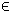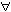## 10MINLP Problem

The mixed-integer nonlinear programming (minlp) problem is defined as
 min x
f(x)

s/t
 −∞ < xL ≤ x ≤ xU < ∞ bL ≤ A x ≤ bU cL ≤ c(x) ≤ cU, xjNjI,
(12)
where x, xL, xURn, f(x)R, ARm1 × n, bL,bURm1 and cL,c(x),cURm2. The variables xI, the index subset of 1,...,n, are restricted to be integers.

The following files define a problem in TOMLAB.

File: tomlab/quickguide/minlpQG_f.m, minlpQG_g.m, minlpQG_H.m, minlpQG_c.m, minlpQG_dc.m, minlpQG_d2c.m
```  f:   Function value
H:   Hessian matrix
c:   Nonlinear constraint vector
d2c: The second part of the Hessian to the Lagrangian function for the nonlinear constraints.
```
The following file illustrates how to solve a MINLP problem in TOMLAB. Also view the m-files specified above for more information.

File: tomlab/quickguide/minlpQG.m

Open the file for viewing, and execute minlpQG in Matlab.
```% minlpQG is a small example problem for defining and solving
% mixed-integer nonlinear programming problems using the TOMLAB format.

Name='minlp1Demo - Kocis/Grossman.';

IntVars   = [ 0 0 1 1 1 ]; % Integer variables: x(3)-x(5)
VarWeight = [ ];           % No priorities given

% There are divisions and square roots involving x(2), so we must
% have a small but positive value for the lower bound on x(2).
BIG = 1E8;

x_L = [ 0   1/BIG   0 0 0 ]';    % Lower bounds on x
x_U = [ BIG  BIG    1 1 1 ]';   % Upper bounds on x

% Three linear constraints
A = [1 0     1  0 0 ; ...
0 1.333 0  1 0 ; ...
0 0    -1 -1 1 ];

b_L = [];               % No lower bounds
b_U = [1.6 ; 3 ; 0];    % Upper bounds

c_L = [1.25;3];         % Two nonlinear constraints
c_U = c_L;              % c_L==c_U implies equality

x_0 = ones(5,1);        % Initial value

x_opt = [1.12,1.31,0,1,1]';  % One optimum known
f_opt = 7.6672;              % Value f(x_opt)

x_min = [-1 -1 0 0 0];  % Used for plotting, lower bounds
x_max = [ 1  1 1 1 1]; % Used for plotting, upper bounds

HessPattern = spalloc(5,5,0);  % All elements in Hessian are zero.
ConsPattern = [ 1 0 1 0 0; ...  % Sparsity pattern of nonlinear
0 1 0 1 0 ];    % constraint gradient

fIP = [];        % An upper bound on the IP value wanted. Makes it possible
xIP = [];        % to cut branches. xIP: the x value giving fIP

% Generate the problem structure using the TOMLAB Quick format
Prob = minlpAssign('minlpQG_f', 'minlpQG_g', 'minlpQG_H', HessPattern, ...
x_L, x_U, Name, x_0, ...
IntVars, VarWeight, fIP, xIP, ...
A, b_L, b_U, 'minlpQG_c', 'minlpQG_dc', 'minlpQG_d2c', ...
ConsPattern, c_L, c_U, ...
x_min, x_max, f_opt, x_opt);
Prob.DUNDEE.optPar(20) = 1;
Prob.P = 1;   % Needed in minlpQG_xxx files

% Get default TOMLAB solver for your current license, for "minlp" problems
% Solver = GetSolver('minlp');

% Call driver routine tomRun, 3rd argument > 0 implies call to PrintResult

Result  = tomRun('minlpBB',Prob,2);```